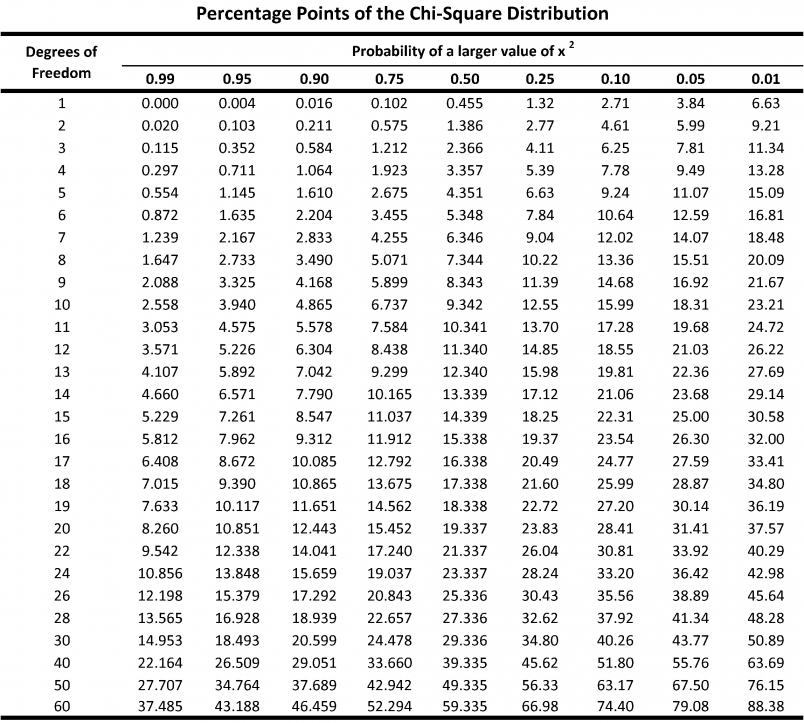# Ch. 14 Chi Squared Test

Sections covered: 14.3

## 14.3 Two-Way Contingency Tables

Skip: pp. 639-643, including “Testing for Homogeneity”

Focus on “Testing for Independence (Lack of Association)”

Notes on the chi square test formula on p. 644:

• Write the null hypothesis as a sentence, not as in the book. (For example: “Class and Survival Status are independent.”)

• “estimated expected” in the textbook is the same as “expected” used in class

• I and J refer to the number of rows and columns in the table

## Resources

Chi Square TableChi Squared Test Calculator

Chi Squared Distribution Curves

## R

The chisq.test() function requires that data be in matrix form:

# p. 647, #28
mat <- matrix(c(28, 17, 7, 31, 26, 10, 26, 19, 11), nrow = 3, byrow = TRUE)

dimnames(mat) <- list(Email_Provider = c("gmail", "Yahoo", "Other"),
Cell_Phone_Provider = c("ATT", "Verizon", "Other"))

chisq.test(mat, correct = FALSE)
##
##  Pearson's Chi-squared test
##
## data:  mat
## X-squared = 1.5074, df = 4, p-value = 0.8253

To see the expected values:

results <- chisq.test(mat, correct = FALSE)
round(results\$expected, 2)
##               Cell_Phone_Provider
## Email_Provider   ATT Verizon Other
##          gmail 25.26   18.42  8.32
##          Yahoo 32.54   23.74 10.72
##          Other 27.20   19.84  8.96

Mosaic plot

mosaicplot(t(mat), color = c("aliceblue", "cornflowerblue", "navyblue"), main = "")See this tutorial for more on mosaic plots.

## Practice Exercises

1. (Class example) We took a survey involving 20 children and 80 adults. 1 of the children and 49 of the adults drink coffee, while the remainder do not. Does there appear to be a relationship between age (child vs. adult) and coffee drinking status (yes vs. no)?

[Ans]

x <- c(1, 49, 19, 31)
dim(x) <- c(2, 2)
x
##      [,1] [,2]
## [1,]    1   19
## [2,]   49   31
chisq.test(x, correct = FALSE)
##
##  Pearson's Chi-squared test
##
## data:  x
## X-squared = 20.25, df = 1, p-value = 0.000006795

1. (Hypothesis Testing) In an investigation of alcohol use among college students, each male student in a sample was categorized both according to age group and according to the number of heavy drinking episodes during the previous 30 days.
18-23 21-23 $$\geq$$ 24
None 357 293 592
1-2 218 285 354
3-4 184 218 185
$$\geq$$ 5 328 331 147

Does there appear to be an association between extent of binge drinking and age group in the population from which the sample was selected? Carry out a test of hypotheses at significance level .01. (Testbook 14.25)

[Ans]

$$α = .01$$

$$H_0$$: the extent of binge drinking and age group are independent

$$H_A$$: the extent of binge drinking and age group are not independent.

data <- c(357, 218, 184, 328, 293, 285, 218, 331, 592, 354, 185, 147)
dim(data) <- c(4, 3)
data
##      [,1] [,2] [,3]
## [1,]  357  293  592
## [2,]  218  285  354
## [3,]  184  218  185
## [4,]  328  331  147
# optional: give names to the rows and columns
dimnames(data) <- list(Episodes = c("None", "1-2", "3-4", ">= 5"),Age Group = c("18-23", "21-23", ">=24"))

data
##         Age Group
## Episodes 18-23 21-23 >=24
##     None   357   293  592
##     1-2    218   285  354
##     3-4    184   218  185
##     >= 5   328   331  147
chisq.test(data, correct = FALSE)
##
##  Pearson's Chi-squared test
##
## data:  data
## X-squared = 212.91, df = 6, p-value < 0.00000000000000022

$$p-value < .01$$

Reject $$H_0$$. There appears to be an association between extent of binge drinking and age group in the population from which the sample was selected.